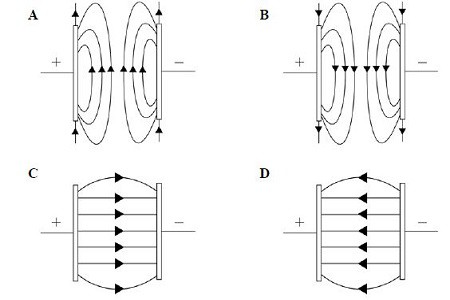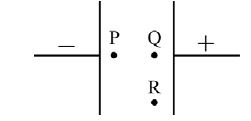Physic Tutorials

# Electric Field Examples And Answers

Electric field is a region of space where a charge experience a force. In this article, I will be providing solution to some electric field examples.

Example 1

Which diagram correctly shows the electric field between two charged plates?the electric field lines between two parallel plates are

• parallel
• equally space
• curve at the edge which is know as edge effect
• the field lines moves from the positive plate to the negative plate

From the above condition, option C is the right answer.

Example 2

The diagram shows two parallel, charged plates.Which statement about the electric field at points P, Q and R is correct?
A The field at P is greater than the field at Q and R.
B The field at P is less than the field at Q and R.
C The fields at P and Q are equal but greater than the field at R.
D The fields at all points P, Q and R are all equal.

Kindly note that the electric field in the above diagram is a uniform electric field i.e. the field that exist between two parallel charged plates.

This then means that the electric field strength at any point in the field is the same. Option D is right.

Example 3

There is a potential difference of 200 V across a pair of parallel plates which are 4.00 cm apart. Calculate the force on a charge of 2.50 nC which is between the plates.

The electric field strength formula = V/d, also, E =f/q

V is the potential difference across the plate

d is the distance between the plate

f is the force on the charge

q is the charge

equating the two formulas,

f/q = v/d

f = vq/d = 200*2.5*10^-9/4*10^-2= 125*10^-6 N = 1.25*10^-5N

Example 4

An isolated metal sphere A of radius 26 cm is positively charged. Sphere A is shown in Fig below.Electrical breakdown (a spark) occurs when the electric field strength at the surface of the sphere exceeds 2.0 × 10^4 V m–1. Calculate the maximum charge Q that can be stored on the sphere. (CIE Physics may/june 2018 P41)

E = Q/(4*pi*permitivity*r^2)

Q = 20000*4*3.142*8.85*10^-12*0.26^2

Q = charge = 1.5 × 10^–7C

Example 5

A particle has a charge of +2.0 mC and is in a vertical uniform electric field. An electric force of 1.0 × 10–2N acts upwards on the particle. What is the electric field strength?
A 0.20 V m–1 downwards
B 0.20 V m–1 upwards
C 5.0 V m–1 downwards
D 5.0 V m–1 upwards